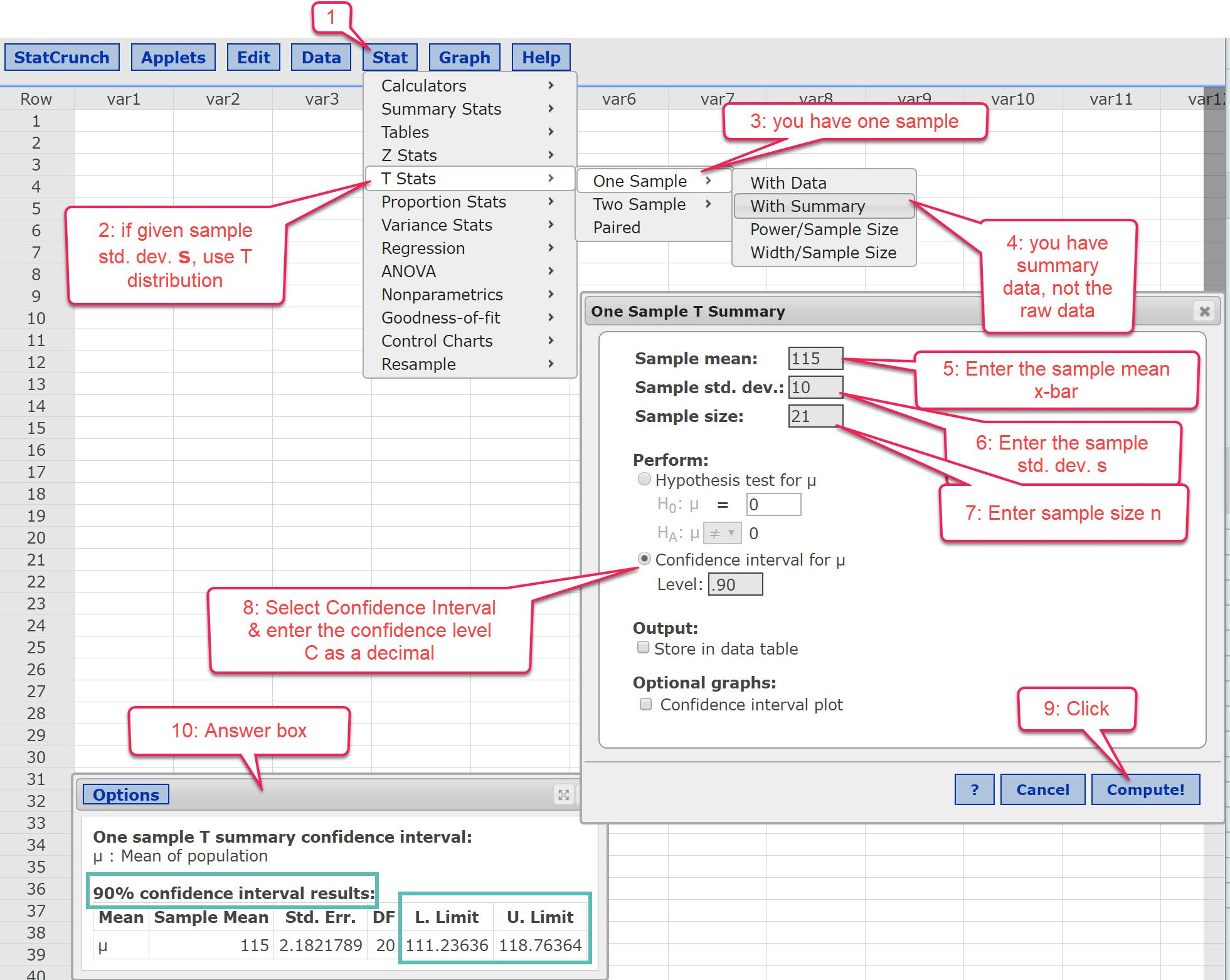# Confidence Interval for a Mean using StatCrunch

If you are given summary data and asked to find a confidence interval, it is relatively easy using StatCrunch. Here is a typical problem:In this problem, you are told to use the t-distribution, but that may not always be the case. If you are given the sample standard deviation, s, instead of the population standard deviation, σ , use the t-distribution to find the confidence interval. In StatCrunch, this is in the T Stats menu. If you are given σ or are told to use the z-distribution, it is essentially the same steps except you select Z Stats in step 2.